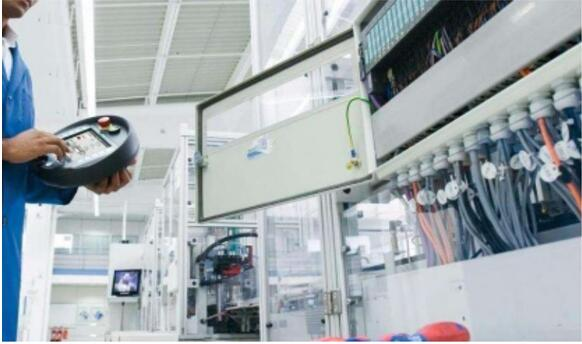EN

### What is Subpixel?

share:

2021/05/04

author:POMEAS

What is Subpixel?

Pixel is the smallest unit of image plane of area array camera. For example, a CMOS camera chip has a pixel spacing of 5.2 microns. The continuous images in the physical world are discretized when the camera is taking pictures. Each pixel on the imaging plane represents only the color near it. To what extent is "near"? It's hard to explain. There is a distance of 5.2 microns between the two pixels, which can be seen as connected macroscopically. But at the micro level, there are infinitely smaller things between them. This smaller thing we call "sub-pixel". In fact, "sub-pixel" should exist, but there is no tiny sensor on the hardware to detect it. So the software calculates it approximately.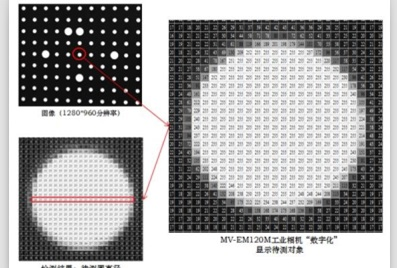Subpixel Accuracy

Subpixel accuracy refers to the subdivision between adjacent two pixels. Input values are usually one-half, one-third or one-quarter. This means that each pixel will be divided into smaller units to implement interpolation algorithms for these smaller units. For example, if a quarter is chosen, it is equivalent to each pixel being calculated as four pixels in both horizontal and vertical directions. Therefore, if a 5x5-pixel image chooses a quarter of the sub-pixel accuracy, it is equivalent to creating a 16x16 discrete lattice, and then interpolating the lattice. Refer to the figure below. The red dot represents the original pixel and the black dot represents the newly generated sub-pixel.

Application of Subpixel in Machine Vision

In machine vision, sub-pixel is a common concept. In many functions, we can choose whether to use sub-pixel or not. In measurement, such as position, line, circle, etc., sub-pixel will appear. For example, the diameter of a circle is 100.12 pixels. The latter 0.12 is the sub-pixel. Because it can be understood from the pixels that the smallest physical unit of industrial camera is actually the pixel, but we can still get the decimal point value in the machine vision measurement, which is calculated by software. In fact, in the real situation, it is not necessarily very accurate. This value is usually more easily reflected in grayscale images, while in binary images, because the value is only 0,1. So many functions do not necessarily compute sub-pixels.

### TECHNICAL SOLUTION

MORE+

You may also be interested in the following information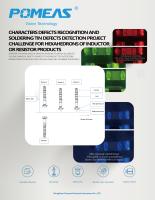### Characters And Soldering Tin Defects Recognition Detection For Hexahedrons Inductor Or Resistor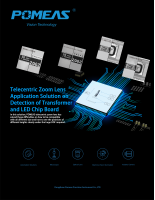### Transformer and LED Chip Board Detection Solution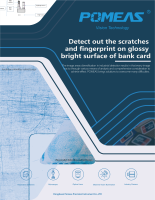### Detect Out The Scratches and Fingerprint On Bank Card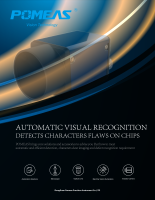### FREE CONSULTING SERVICE

Let’s help you to find the right solution for your project!